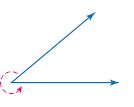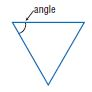Homework Explained - Math Practice 101Dear guest, you are not a registered member. As a guest, you only have read-only access to our books, tests and other practice materials.

As a registered member you can:

Registration is free and doesn't require any type of payment information. Click here to Register.
Go to page:
Chapter 7: Geometric Figures; Lesson 1: Classify Angles

• Question 12

Angles ABC and DBE are vertical angles. If the measure of $$\angle ABC$$ is 40°, what is the measure of $$\angle ABD$$?

• Type below:

### H.O.T. Problems Higher Order Thinking

• Question 13

Model with Mathematics Draw examples of angles that represent real-world objects. Be sure to include at least three of the following angles: acute, right, obtuse, straight, vertical, and adjacent. Verify by measuring the angles.

• Type below:
• Question 14

Reason Inductively Explain how you can use a protractor to measure the angle shown. Find the measure of the angle.• Type below:

Persevere with Problems Determine whether each statement is true or false. If the statement is true, provide a diagram to support it. If the statement is false, explain why.

• Question 15

A pair of obtuse angles can also be vertical angles.

• Question 16

A pair of straight angles can also be adjacent angles.

• Question 17

Which word best describes the angle marked in the figure?• A. acute
• B. obtuse
• C. right
• D. straight

Yes, email page to my online tutor. (if you didn't add a tutor yet, you can add one here)